On the meadow grazing horses, cows, and sheep, together with less than 200. If cows were 45 times more, horses 60 times more, and sheep 35 times more than there are now, their numbers would equally. How many
horses, cows, and sheep are on the meadow together?

x1 =  85
x2 =  170

### Step-by-step explanation:

x = a+b+c < 200
b = 45/60 a
c = 45/35 a
a + 45/60 a + 45/35 a < 200
LCD(11, 4560, 4535) = LCM(1, 60, 35) = 22×3×5×7 = 420
11 = 420420
4560 = 315420
4535 = 540420

420 k + 315 k + 540 k < 84000
1275 k < 84000
17 k < 1120

x = 56+42+72 = 170
x = 28+21+36 = 85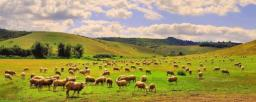Did you find an error or inaccuracy? Feel free to write us. Thank you!Tips to related online calculators
Do you want to calculate least common multiple two or more numbers?
Need help to calculate sum, simplify or multiply fractions? Try our fraction calculator.

## Related math problems and questions:

• Lord Ram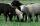When lord Ram founded the breed white sheep was 8 more than black. Currently white sheep are four times higher than at the beginning and black three times more than at the beginning. White sheep is now 42 more than the black. How many white and black shee
• AthletesAthletes at the stadium could enter two-steps, three-steps, four-steps, five-steps, six-steps. There were more than 100 but less than 200. How many athletes were there?
• Fewer than 500 sheep,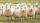There are fewer than 500 sheep, but if they stand in a double, triple, quadruple, five, and sixth-order, one sheep will remain. But they can stand in the seventh order. How many are sheep?
• School year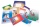At the beginning of the school year, 396 notebooks and 252 textbooks are ready to be distributed in the classroom. All pupils receive the same number of notebooks and the same amount of textbooks. How many pupils are there in the class if you know that th
• Dinosaurs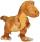More than 30 and less than 60 dinosaurs have met at the pond. A quarter of them bathed and 1/7 saws, and the rest gripped. How many were at the pond? How many were there?
• Sheep and cowsThere are only sheep and cows on the farm. Sheep is eight more than cows. The number of cows is half the number of sheep. How many animals live on the farm?
• Four poplarsFour poplars are growing along the way. The distances between them are 35 m, 14 m, and 91 m. At least how many poplars need to be dropped to create the same spacing between the trees? How many meters will it be?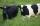Grass grows in the meadow equally fast and evenly. It is known that 99 cows graze meadow for 14 days and 95 cows by 22 days. How many cows graze meadow for 77 days?
• Clock's gears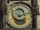In the clock machine, three gears fit together. The largest has 168 teeth, the middle 90 teeth, and the smallest 48 teeth. The middle wheel turns around its axis in 90 seconds. How many times during the day do all the gears meet in the starting position?
• Reminder and quotientThere are given numbers A = 135, B = 315. Find the smallest natural number R greater than 1 so that the proportions R:A, R:B are with the remainder 1.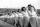Practitioners lined up in rectangle with row with four, five or six exercisers, one always missing to full rectangle. How many exercisers were on the field, if they have estimated not been more than 100?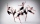4 dance ensembles were dancing at the festival. None had less than 10 and more than 20 members. All dancers from some of the two ensembles were represented in each dance. First, 31 participants were on the stage, then 32, 34, 35, 37, and 38. How many danc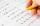9.A to attend more than 20 students but fewer than 40 students. A third of the pupils wrote a math test to mark 1, the sixth to mark 2, the ninth to mark 3. No one gets mark 4. How many students of class 9.A wrote a test to mark 5?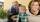Grandson with grandpa, they counted how many years have together. Their product is 365. How many years is the sum of their years?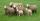Shepherd tending the sheep. Tourists asked him how much they have. The shepherd said, "there are fewer than 500. If I them lined up in 4-row 3 remain. If in 5-row 4 remain. If in 6-row 5 remain. But I can form 7-row." How many sheep have herdsman?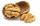How many we must have at least nuts if we can equally divide it to 10 children, 12 children or 15 children and any nut left?Find the smallest multiple of 63 and 147